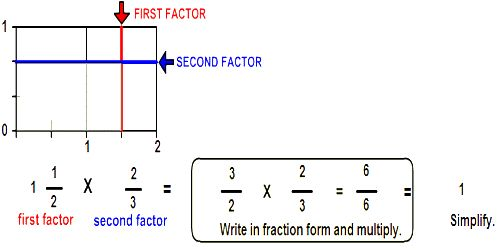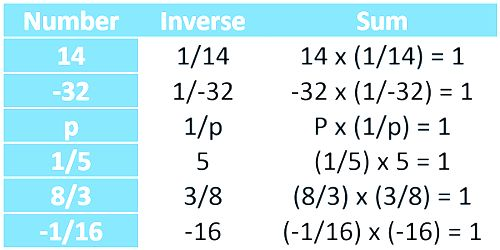Mathematic

# Reciprocal or Multiplicative InverseReciprocal or Multiplicative Inverse

When the product of two numbers is one, they are called reciprocals or multiplicative inverses of each other. For example, 2/7 and 7/2 are reciprocals because (2/7 x 2/7) = 14/14 = 1. This is the motivation for the following property of fractions. The reciprocal of x is 1/x. In other words, a reciprocal is a fraction flipped upside down. Multiplicative inverse means the same thing as reciprocal.

If the product of two numbers is 1, then each number is known as multiplicative inverse or the reciprocal of one another.

The following are the rules of multiplicative inverse of a fractional number:

(a) To find the multiplicative inverse of a proper or improper fraction, interchange the numerator and denominator.

(b) To find the reciprocal of a mixed fraction or whole number, first change it into an improper fraction, then interchange the numerator and denominator.

Multiplicative inverse is also known as reciprocal.Example:

The product of a number and its reciprocal equals 1.

The reciprocal of 4 is 1/4.

The reciprocal of 2/3 is 3/2.

The reciprocal of 1 is 1.

The number 0 does not have a reciprocal because the product of any number and 0 equals 0.

The reciprocals of a number is sometimes called the Multiplicative Inverse of the number.

The product of a negative number and its reciprocal equals 1. If the number is negative then the reciprocal must also be negative to produce a product of +1.

The reciprocal of -4 is -1/4.

The reciprocal of -2/3 is -3/2.

The reciprocal of -1 is -1.

Information Source: